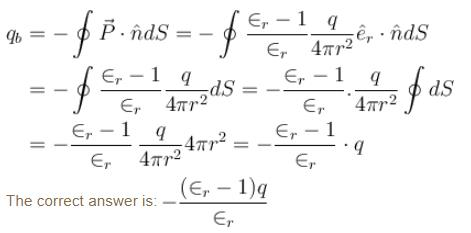Courses

# Polarisation Of Dielectrics MCQ Level - 2

## 10 Questions MCQ Test Basic Physics for IIT JAM | Polarisation Of Dielectrics MCQ Level - 2

Description
This mock test of Polarisation Of Dielectrics MCQ Level - 2 for Physics helps you for every Physics entrance exam. This contains 10 Multiple Choice Questions for Physics Polarisation Of Dielectrics MCQ Level - 2 (mcq) to study with solutions a complete question bank. The solved questions answers in this Polarisation Of Dielectrics MCQ Level - 2 quiz give you a good mix of easy questions and tough questions. Physics students definitely take this Polarisation Of Dielectrics MCQ Level - 2 exercise for a better result in the exam. You can find other Polarisation Of Dielectrics MCQ Level - 2 extra questions, long questions & short questions for Physics on EduRev as well by searching above.
QUESTION: 1

### A plain slab of isotropic material of relative permittivity ∈r = 5 is placed normal to a uniform electric field with an electric displacement vector of magnitude 5 Cm2. If the slab occupies a volume of 0.5m3 and is uniformly polarized. Then magnitude of total dipole moment of the slab.

Solution: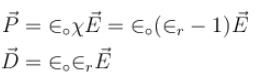⇒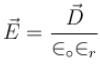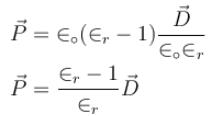Here represent the average electric field inside the dielectric. Also the magnitude of total dipole moment of the slab.
ptotal  = P × volume of the slab
= 4 × 0.5 = 2Cm

QUESTION: 2

### A point charge q is located in vacuum at a distance l from the plane surface of a uniform isotropic dielectric filling up all the half space. If the permittivity of the dielectric equal to ∈. Then the surface density of bound charges.

Solution:

Let ρ is the surface density of bound charge. Since, there is no free charge at interface.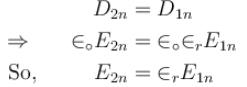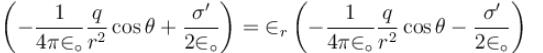Where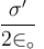is the field produced by bound charges near the dielectric vaccum interface.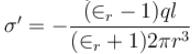The correct answer is: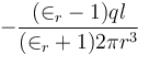QUESTION: 3

### The radii of the inner and outer spheres of a condenser are 9cm and 10cm respectively. If the dielectric constant of the medium between the two sphere is 6 and the charge on the inner sphere is 18 × 10–9C, then the potential of inner sphere will be, if the outer sphere is earthed

Solution:

Given system is a spherical capacitor. So, capacitance of system.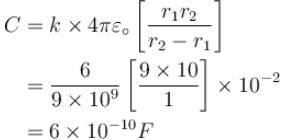Now, potential of inner sphere will be equal to potential difference of the capacitor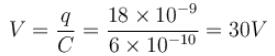The correct answer is: 30 V

QUESTION: 4

The capacitance of a parallel plate capacitor with air as medium is 3µF, with introduction of a dielectric  medium between the plates, the capacitance becomes 15µF. The permitting of the medium is

Solution:

Capacitance of a parallel plate capacitance with air is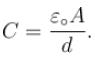Capacitance of a same parallel plate capacitor with the introduction of a dielectric medium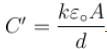,  where k is the dielectric constant of the medium. Dielectric constant is given as :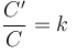⇒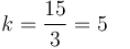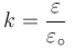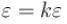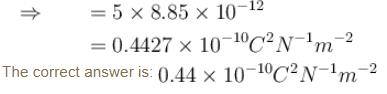QUESTION: 5

The plates in a parallel plates capacitor are separated by a distance d with air as the medium between the plates. In order to increase the capacity by 66%, a dielectric slab of dielectric constant 5 is introduced  between the plate. What is the thickness of dielectric slab.

Solution:

The capacity in air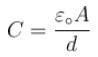The capacity when dielectric slab of dielectric constant 5 is introduced between the plates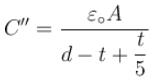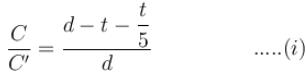Capacity is increased by 66%
So, new capacity :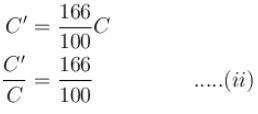From (i) and (ii)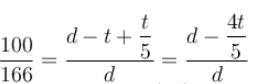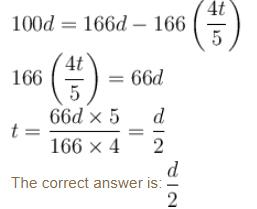QUESTION: 6

A dielectric sphere of radius R carries polarization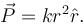Where r is the distance from the centre and k is a constant. In the spherical polar coordinate system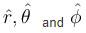are unit vectors. The  volume  bound charge density and electric field respectively inside the sphere at a distance d from the centre is

Solution: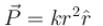Now, volume charge density of bound charge,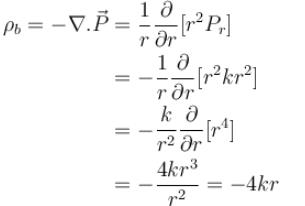Volume charge density at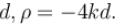Since, the sphere is statically polarized.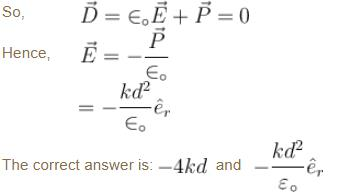QUESTION: 7

Figure shows a dielectric-conductor interface. If ∈r is the permittivity of dielectric and ρ is the surface charge density of the charge on the conductor then the surface charge density of the dielectric’s bound charge.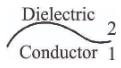Solution:

If ρ is the surface density of free charges at the conductor dielectric interface.
Applying boundary condition of D vector.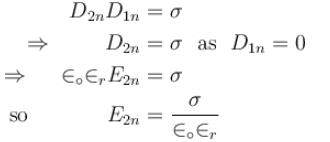Applying boundary condition of polarization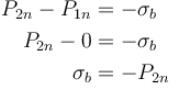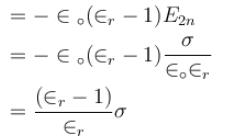So, surface density of the bound charge at the conductor dielectric interface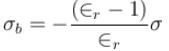The correct answer is: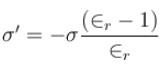QUESTION: 8

The plate of a parallel plate condenser are pulled apart with velocity v. If at any instant their mutual distance of separation is d, then the magnitude of the time rate of change of capacity depend on d as follows :

Solution:

Capacity of a condenser is given as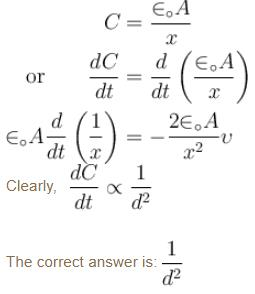QUESTION: 9

Figure shows four plates each of area A and separated from one another by a distance d. The capacitance between A and B is.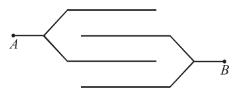Solution:

The four plates are alternately connected. They form three capacitors in parallel.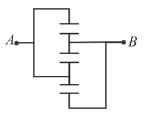The capacity of each capacitor is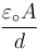So, net capacity is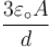The correct answer is: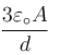QUESTION: 10

A point charge q is located at the centre of a ball made of a uniform isotropic dielectric with relative permittivity ∈r. Then total amount of bound charge enclosed by a sphere whose radius is less than the radius of the ball.

Solution:

The free charge located at the centre of sphere will produce an electric field which will polarize the dielectric sphere.
Polarization of dielectric will produce bound charges.
Using Gauss’s law.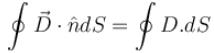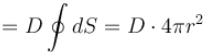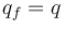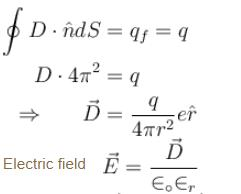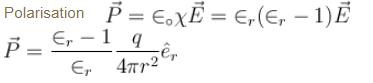Amount of bound charge enclosed by Gaussian surfaces